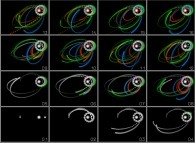# Real Numbers Do Not Exist for a Computer

####Jean-François COLONNA www.lactamme.polytechnique.frjean-francois.colonna@polytechnique.edu CMAP (Centre de Mathématiques APpliquées) UMR CNRS 7641, Ecole Polytechnique, CNRS, 91128 Palaiseau Cedex, France france telecom, France Telecom R&D

[Site Map, Help and Search [Plan du Site, Aide et Recherche]]
[The Y2K bug [Le bug de l'an 2000]]
[Do you believe that Real Numbers exist for a computer and that floating point computations are safe?]
[Please, visit A Virtual Machine for Exploring Space-Time and Beyond, the place where you can find thousands of pictures and animations between Art and Science]
(CMAP28 WWW site: this page was created on 03/18/1996 and last updated on 01/28/2014 15:14:08 -CET-)

• There is an infinity of real numbers, each of which is mathematically represented by a infinite sequence of digits.

• A computer has a finite capacity. It can only represent a finite set of real numbers, each of which by a finite sequence. This introduces a rounding-off error in this representation as well as in the results of subsequent operations.

• Everybody knows that associativity holds for additions and multiplications on real numbers. Because of rounding-off, the order in which the operations are performed is important in a computer, and associativity fails; for most values A, B and C:
```                    (AxB)xC # Ax(BxC)
```
(this is also true for the addition, but the effect on the multiplication is worse).

• This can be seen with non linear iterative processes. Let's see what happens with the Verhulst dynamics:
```                                       2
X  = (R+1)X    - RX
n         n-1     n-1
```
We can rewrite this expression in five different mathematically equivalent ways.
```                    X  = X    + (R(X    - (X   X   )))
n    n-1       n-1     n-1 n-1

X  = (RX   ) + ((1 - (RX   ))X   )
n      n-1             n-1   n-1

X  = (((R+1) - (RX   ))X   )
n                n-1   n-1

X  = ((R+1)X   ) - ((RX   )X   )
n          n-1        n-1  n-1

X  = ((R+1)X   ) - (R(X   X   ))
n          n-1        n-1 n-1
```
Let's compare the results of these five different formulations on a computer.

Such astonishing differences could appear in any program as soon as:

• -the user moves the parentheses,
• -the user changes the system and compiler releases,
• -the user changes the compiling options,
• -the instruction scheduling is disturbed,
• -the floating point processor and the internal format of numbers are changed,
• -etc...

• The concrete N-body problem will help us visualize how wrong numerical results obtained with a computer can be. N bodies are gravitationally interacting and evolve according to Newton laws; each body is subject to the following acceleration:
```
2 ---->    i=N
d   OC     -----       M
k    \            i     ----->
--------- = /     G ----------- C C
2      -----            3   k i
dt        i=1     |----->|
(i#k)    | C C  |
k i
```

• These equations are chaotic as soon as N is greater than 2, and can only be solved numerically in order to give the positions of the bodies as a function of time. We are going to use the very same C-program on three different computers which we call Red, Green and Blue. Let's start the program on the computers with the same initial conditions. Devastating differences again appear. At first, the three computers give the same values, and the superposed bodies appear white. Unfortunately, this does not last long: slowly and then faster and faster, the predictions of the three computers become absolutely different: the Red computer forecasts the ejection of a body, but not the other two.

• The major difference between the machines lies in their compilers which do not translate in the same way the C statements into assembly instructions. The operations are not performed in the same order, and the lack of associativity causes this phenomenon!

• Very complex non-linear models are used in Science and Industry, such as those for weather forecasting. They can only be studied on computers and are subject to the same phenomenon. studied.

• What to do next?

• And God says:
```                    cd        /Universe
rm        -rf *
reboot
```